# 2nd PUC Chemistry Question Bank Chapter 2 Solutions

## Karnataka 2nd PUC Chemistry Question Bank Chapter 2 Solutions

### 2nd PUC Chemistry Solutions NCERT Textbook Questions and Answers

Question 1
Define the term solution. How many types of solutions are formed? Write briefly about each type with an example.
A true solution is a homogenous mixture of two or more substances. The constituent . particle which is in larger amount’’ is called a solvent and that in smaller quantity is called a solute.

Since the solvent ans solute may be either . gaseous, liquid and solid, the number of possible types of binary solutions than can be prepared are given below.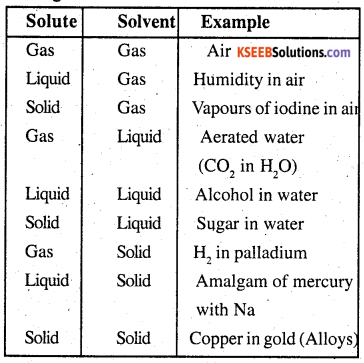Question 2.
Suppose a solid solution is formed are very small. What kind of solid solution is this likely to be?
Solid in solid type. E.g: Copper in gold. This type of solutions are called alloys.

Question 3.
Define the following terms:
(i) Mole fraction
(ii) Molality
(iii) Molarity
(iv) Mass percentage.
(i) Mole fraction (X): The mole fraction of any component in a solution is the ratio of the number of moles of that component to the
sum of the number of moles of all the components present in the solution.
For a binary solution containing A and B, Mole fraction of A,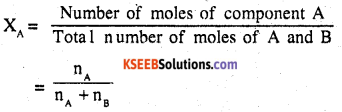where nA and nB are the numbers of moles of components A and B respectively.

(ii) Molality (m): Molality is the numberof moles of the solute dissolved in 1000 gms (1 kg) of the solvent. It B denoted by ‘m’ mathematically.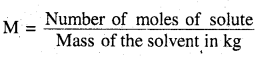(iii) Molarity of a solution is defined as the number of moles of the solute dissolved per litre (or dm3) of solution. It is denoted by ‘M’ mathematically.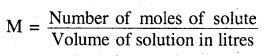(iv) Mass fraction multiplied by 100 gives mass percentage. E.g.: mass percentage of A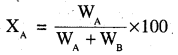Question 4.
Concentrated nitric acid used in laboratory work is 68% nitric acid by mass in aqueous solution. What should be the molarity of such a sample of the acid if the density of the solution is 1.504 g mLr-1?
68% of nitric acid by mass means that
Mass of nitric acid = 68g
Mass of solution = 100g
Molar mass of HN03 = 63g mol-1?
∴ 68 g HNO3 = $$\frac{68}{63}$$ mole = 1.079 mole
Density of solution = 1.504g mL-1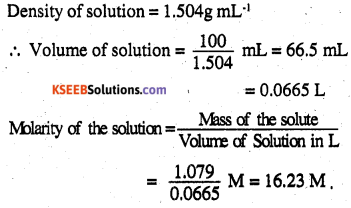Question 5.
A solution of glucose in water is labelled as 10% w/w, what would be the molality and mole fraction of each component in the solution? If the density of solution is 1.2 g mL-1, then what shall be the molarity of the solution?
10 g glucose is present in 100 g solution, i.e., 90 g of water = 0.090 kg of H2O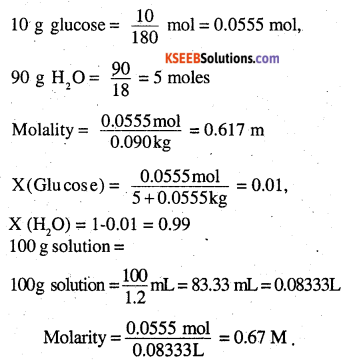Question 6.
How many mL of 0.1 M HCl are required to react completely with 1 g mixture Of Na2CO3 and NaHC03 containing equimolar amounts of both?
Step 1: To calculate the number of moles of the components in mixture
suppose Na2CO3 present in the mixture = X g ,
NaHCO3 present in the mixture = (1 – x) g
Molar mass of Na2CO3 = 2 x 23+12+3 x 16=106g mol-1
Molar mass of NaHCO3.
= 23 + 1 + 12 + 3 × 16 = 84g mol-1
∴  Moles of Na2CO3 in xg = $$\frac{ x }{ 106}$$
Moles of NaHCO3 in (1-x) g = $$\frac{1-x}{84}$$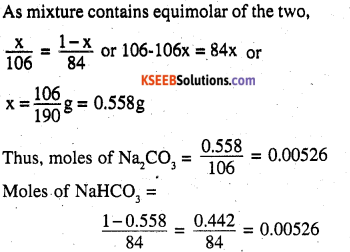Step 2: To calculate the moles of HC1 required.
Na2CO3 + 2 HCl → 2 NaCl + H2O + CO2
NaHCO3 + HCl → NaCl + H2O + CO2
1 mole of Na2CO3 required HCl = 2 moles
0.00526 mole of Na2CO3 requires HCl
= 0.00526 × 2 moles = 0.01052
1 mole of NaHCO3 required HCl = 1 mole
0.00526 mole of NaHCO3 required HCl
= 0.00526 mole
∴Total HCl required = 0.01052 + 0.00526
=0.01578 moles

Step3: To calculate volume of 0.1M HCl
0.1 mole of 0.1 M HCl are present in 1000 mL of HCl
0.01578 mole of 0.1 M HCl will be present in HCl = $$\frac{1000}{0.1}$$ x 0.01578
= 157.8 ml.

Question 7.
A solution is obtained by mixing 300 g of 25% solution and 400 g of 40% solution by mass. Calculate the mass percentage of the resulting solution.
300 g of 25% solutions contains solute = 75g
400 g of 40% solution contains solute = 160 g.
Total solute = 160 + 75 = 235 g
Total solution=300 + 400 = 700 g
% of solute in final solution = $$\frac{235}{700}$$ × 100 = 33.5%
% of water in the final solution = 100 – 33.5 = 66.5%Question 8.
An antifreeze solution is prepared from 222.6 g of ethylene glycol (C2H6O2) and 200 g of water. Calculate the molality of the solution. If the density of the solution is 1.072 g mL-1, then what shall be the molarity of the solution?
Mass of the solute, C2H4(OH)2 = 222.6g
Molar mass of C2H4 (OH)2 = 62 g mol-1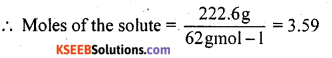Mass of the solvent = 200 g = 0.200 kg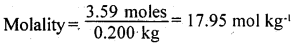Total mass of the solution = 422.6g
Volume of the solutions =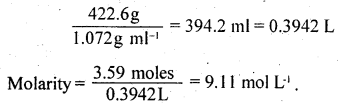Question 9.
A sample of drinking water was found to be severely contaminated with chloroform (CHCl3) supposed to be a carcinogen. The level of contamination was 15 ppm (by mass):
(i) express this in percent by mass
(ii) determine the molality of chloroform in the water sample.
15 ppm means 15 parts in million (106) parts by mass in solution
∴ % by mass = $$\frac{15}{10^{6}} \times$$ × 100=15 × 10-4
Taking 15 g chloroform in 106g
Molar mass of CHCl3 = 12 + 1 + 3 × 35.5 = 119.5 g mol-1
Molality = $$\frac{15 / 119.5}{10^{6}}$$ × 1000= 1.25 × 10-4 m

Question 10.
What role does the molecular interaction play in a solution of alcohol and water?
There is strong hydrogen bonding in alcohol molecules as well as water molecules. On mixing the molecular interactions are weekend. Hence, they show positive deviations from ideal behaviour. AS a result, the solution will have higher vapour pressure and lower boiling point than that of water and alcohol.

Question 11.
Why do gases always tend to be less soluble in liquids as the temperature is raised?
Dissolution of gas in liquid in an exothermic process (Gas + solvent ⇌ solution + Heat). As the temperature is increased, equilibrium shifts backward.

Question 12.
State Henry’s law and mention some important applications?
Henry’s law states that at constant temperature, the solubility of a gas in a liquid is directly proportional to the pressure of the gas.
Applications: It is used

• in the production of carbonated beverages
• in the deep sea diving
• in the function of lungs.Question 13.
The partial pressure of ethane over a solution containing 6.56 x 10-3 g of ethane is 1 bar. If the solution contains 5.00 x 10-2 g of ethane, then what shall be the partial pressure of the gas?
Applying the relationship m = KH x p
In the first case, 6.56 x 102 g bar-1
In the second case, 5.00 x 10-2 g = (6.56 × 10-2 gbar-1) × p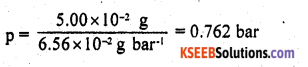Question 14.
What is meant by positive and negative deviations from Raoult’s law and how is the sign of ΔmixH related to positive and negative deviations from Raoult’s law?
In +ve deviation, A-B interactions are weaker than those between A-A or B-B. In such molecules, A or B will find it easier to escape than in pure state. This increases vapour .pressure. In case of -ve deviation, A-B interaction = A-A or B-B. This leads to decrease in vapour pressure.

In +ve deviation, ΔmixH is + ve
In -ve deviation, ΔmixH is – ve

Question 15.
An aqueous solution of 2% non-volatile solute exerts a pressure of 1.004 bar at the normal boiling point of the solvent. What is the molar mass of the solute?
Vapour pressure of pure water at boiling
point (P°) = 1 atm = 1.013 bar
vapour pressure of solution (ps) = 1.004 bar
Mass of solute = (w2) = 2g
Mass of solution = 100 g
Mass of solvent = 98g
Applying Roault’s law for dilute solution (being 2%)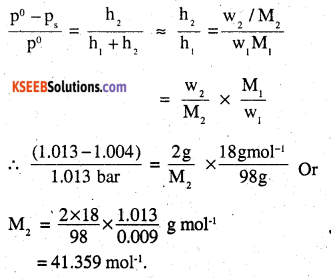Question 16.
Heptane and octane form an ideal solution. At 373 K, the vapour pressures of the two liquid components are 105.2 kPa and 46.8 kPa respectively. What will be the vapour pressure of a mixture of 26.0 g of heptane and 35 g of octane?
Molar mass of heptane (C7 H16) = 100 g mol-1
Molar mass of octane (C8H18) = 114 g mol-1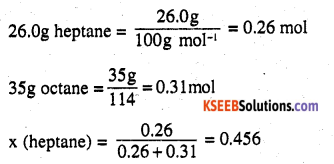x (octane) = 1 – 0.456 = 0.544
p (heptane) = 0.456 x 105.2 kPa = 47.97 kPa
p (octane) = 0.544 x 46.8 kPa = 25.46 kPa
p total = 47.97 + 25.46 = 73.43 kPa

Question 17.
The vapour pressure of water is 12.3 kPa at 300 K. Calculate vapour pressure of 1 molal solution of a non-volatile solute in it.
1 molal solution means 1 mol of the solute in 1 kg of solvent (water)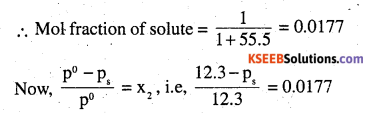or ps = 12.08 kPa

Question 18.
Calculate the mass of a non-volatile solute (molar mass 40 g mol-1) which should be dissolved in 114 g octane to reduce its vapour pressure to 80%.
Ps = 80% of p° = 0.80 p° solute = $$\frac{w}{40} r$$ mol solvent (octane) =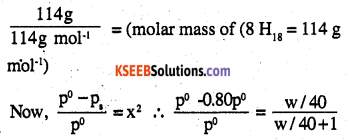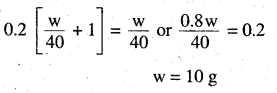Question 19.
A solution containing 30 g of non-volatile solute exactly in 90 g of water has a vapour pressure of 2.8 kPa at 298 K. Further, 18 g of water is then added to the solution and the new vapour pressure becomes 2.9 kPa at 298 K. Calculate:
(i) molar mass of the solute
(ii) vapour pressure of water at 298 K.
Suppose the molar mass of the solute = Mg mol-1
n2 (solute) = $$\frac{30}{M}$$ moles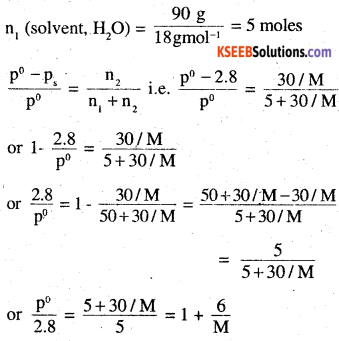After adding 18 g of water,
n(H2O)i.e, n1 = 6 moles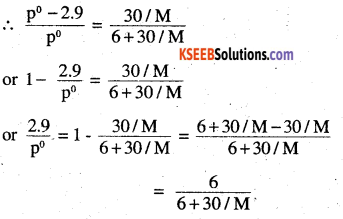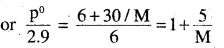Dividing equation (i) by equation (ii), we get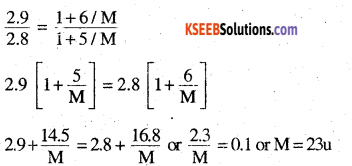(ii) putting M = 23 in equation (i), we get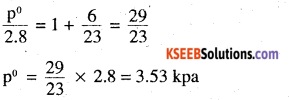Question 20.
A 5% solution (by mass) of cane sugar in water has freezing point of 271 A. Calculate the freezing point of 5% glucose in water if freezing point of pure water is 273.15 K.
Molality of sugar solution =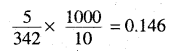∆Tf for sugar solution = 273.15 – 271 = 2.150
∆Tf = Kf × m ∴ Kf = 2.15/0.146
Molality of glucose solution =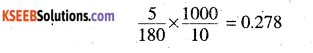Freezing point of glucose solution = 273.15 – 4.09 = 269.06 kQuestion 21.
Two elements A and B form compounds having formula AB2 and AB4. When dissolved in 20 g of benzene (C6H6), 1 g of AB2 lowers the freezing point by 2.3 K whereas 1.0 g of AB4 lowers it by 1.3 K. Tin molar depression – constant for benzene is 5.1 K kg mol-1. Calculate atomic masses of A and B.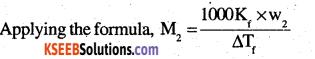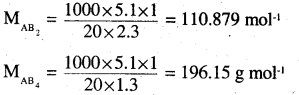Suppose atomic masses of A and B are ‘a’ and ‘b’ respectively. Then
Molar mass of AB2 = a + 2b = 110.87 g mol-1
Molar mass of AB4 = a + 4b =196.15 g mol-1
Equation (ii) – Equation (i) gives
2b = 85.28 orb = 42.64
substituting in equation (i) we get
a + 2 × 42.64 = 110.87 or a = 25.59
Thus atomic mass A = 25.59 u
Atomic mass of B = 4.64 u.

Question 22.
At 300 K, 36 g of glucose present in a litre of its solution has an osmotic pressure of 4.98 bar. If the osmotic pressure of the solution is 1.52 bars at the same temperature, what would be its concentration?
π = CRT
∴ In the first case,
4.98 = $$\frac{36}{180}$$ × R × 300 = 60R
In the second case, 1.52 = C × R × 300
Dividing (ii) by (i), we get C = 0.061 M.

Question 23.
Suggest the most important type of intermolecular attractive interaction in the following pairs.
(i) n-hexane and n-octane
(ii) I2 and CCl4
(iii) NaClO4 and water
(iv) methanol and acetone
(v) acetonitrile (CH3CN) and acetone (C3H6O).
(i) Both are non-polar. Hence, inter molecular interactions in them will be London/ dispersion forces (discussed in class XI)
(ii) Both are non-polar. Hence, inter molecular interactions in them will be London/ dispersion forces (discussed in class XI)
(iii) NaClO4 gives Na+ and ClO4 ions in the solution while water is polar molecule. Hence, intermolecular interactions in them will be ion – dipole interactions.
(iv) Both are polar molecules. Hence intermolecular interactions in them will be dipole-dipole interactions.
(v) Both are polar molecules. Hence intermolecular interactions in them will be dipole-dipole interactions.

Question 24.
Based on solute-solvent interactions, arrange the following in order of increasing solubility in n*octane and explain. Cyclohexane, KCl, CH3OH, CH3CN.
(i) Cyclohexane and n-octane both are non-polar. Hence they mix completely in all proportions.
(ii) KCl is an ionic compound while n-octane is nonpolar. Hence, KCl will not dissolve at all in n-octane.
(iii) CH3OH and CH3CN both are polar but CH3CN is less polar than CH3OH. As the solvent is non-polar, CH3CN will dissolve more than CH3OH is n-octane.
Thus the order of solubility will be KCl< CH3OH < CH3CN < Cyclohexane.

Question 25.
Amongst the following compounds, identify which are insoluble, partially soluble and highly soluble in water?
(i) phenol
(ii) toluene
(iii) formic acid
(iv) ethylene glycol
(v) chloroform
(vi) pentanol.
(i) Partially soluble (because phenol has
polar-OH group but aromatic phenyl, C6H5 – group)
(ii) Insoluble because toluene is non polar while water is polar
(iii) Highly soluble because formic acid can form hydrogen bonds with water.
(iv) Highly soluble because ethylene glycol can form hydrogen bonds with water
(v) Insoluble chloroform is an organic liquid
(vi) Partially soluble because-OH group is polar but the large hydrocarbon part (C5H11) is nonpolar.Question 26.
If the density of some lake water is 1.25g mL-1 and contains 92 g of Na+ ions per kg of water, calculate the molality of Na+ ions in the lake.
Number of moles in 92 g of Na+ ions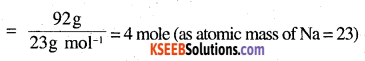As these are present in 1 kg of water, by definition, molality = 4m.

Question 27
If the solubility product of CuS is 6 × 10-16, calculate the maximum molarity of CuS in aqueous solution.
Maximum molarity of CuS in aqueous solution = solubility of CuS in mol L-1
If S in the solubility of CuS in mol L-1 , then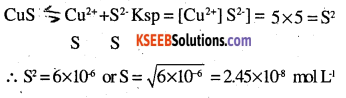Question 28.
Calculate the mass percentage of aspirin (C9H8O4) in acetonitrile (CH3CN) when 6.5 g of C9H8O4 is dissolved in 450 g of CH3CN.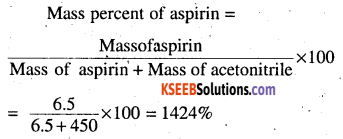Question 29.
Nalorphene (C19H21NO3), similar to morphine, is used to combat withdrawal symptoms in narcotic users. Dose of nalorphene generally given is 1.5 mg. Calculate the mass of 1.5 x 10-3 m aqueous solution required for the above dose.
1.5 × 10-3 m solution means that 1.5 × 10-3 mole of nalorphene is dissolved in I kg of water.
Molar mass of C19H21NO3 = 19 × 12 + 21 + 14 + 48
= 3119 mol-1
∴ 1.5 × 10-3 mole of C19H21NO3;
= 1.5 × 10-3 × 3119 = 0.467 g = 467 mg
∴ Mass of solution = 1000 g + 0.467 g ‘
=1000.467g
Thus, for 467 mg of nalorphene, solution required =1000.467 g for 1.5 mg of nalorphene, solution required =$$\frac{1000.467}{467} \times 1.5 = 3.219$$

Question 30.
Calculate the amount of benzoic acid (C6H5COOH) required for preparing 250 mL of 0.15 M solution in methanol.
0.15 M solution means that 0.15 mole of benzoic acid is present in 1 L
i.e. 1000 mL of the solution.
Molar mass of benzoic acid (C6H5COOH) = 72 + 5 + 12 + 32 + 1 =122 g mol-1
∴ 0.15 mole of benzoic acid = 0.15 × 122g= 18.39
Thus, 1000 mL of the solution contain benzoic acid = 18.39
∴ 250 ml of the solution will contain benzoic acid = $$\frac{18.3}{1000}$$ × 250= 4.575gQuestion 31.
The depression in freezing point of water observed for the same amount of acetic acid, trichloroacetic acid and trifluoroacetic acid increases in the order given above. Explain briefly.
The depression in freezing points are in the order:
acetic acid < trichloroacetic acid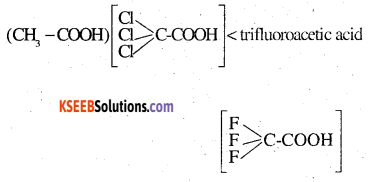Fluorine, being most electronegative, has the highest electron withdrawing inductive effect. Consequently, trifluoroacetic acid is the Strongest acid while acetic acid is the weakest acid. Hence, trifluoroacetic acid ionizes to the largest extent while acetic acid ionizes to minimum extent to give ions.in their.solutions . in water. Greater the ions produced, greater is the depression in freezing point. Hence the depression in freezing point is maximum for fluoroacetic acid and minimum for acetic acid.

Question 32.
Calculate the depression in the freezing point of water when 10 g of CH3CH2 CHCICOOH is added to 250 g of water. Ka = 1.4 × 10-3, Kf= 1.86 K kg mol-1.
Molar mass of CH3CH2CHCICOOH = 15 + 14 + 13 + 35.5 + 45 = 122.5 g mol-1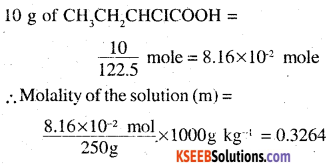If α is the degree of dissociation of CH3CH2CHCICOOH, then CH3CH2CHCLCOOH □ CH3CHXHCICOO + H +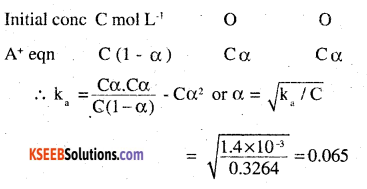To calculate vant’s Hoff factor:
CH3CH2CHCICOOH □ CH3CH2HCICOO + H+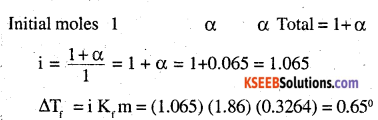Question 33.
19.5 g of CH2FCOOH is dissolved in 500 g of water. The depression in the freezing point of water observed is 1.0°C. Calculate the van’t Hoff factor and dissociation constant of fluoroacetic acid. Kf of water is 1.86 K kg mol-1.
Here, w2 = 19.5 g, w1, = 500g, Kf = 1.86 K kg mol-1, (ATf) obs = 1.0°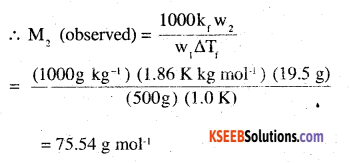M2 (Calculated) for CH2 FCOOH = 14+19+45 = 78 g mol-1
Vant Hoff factor (i) =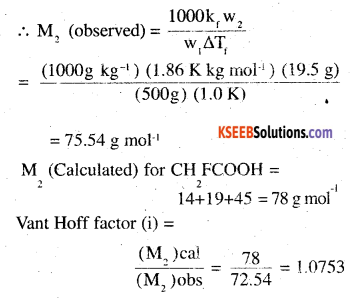calculation of dissociation constant. Suppose degree of dissociation at the given concentration is a.
Then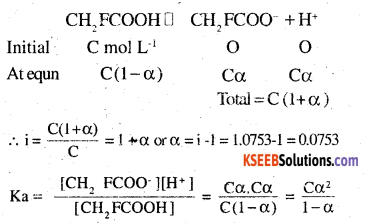Taking volume of the solutions as 500ml,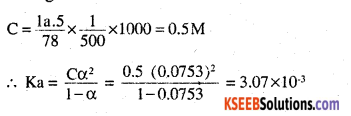Question 34.
Vapour pressure of water at 293 K is 17.535 mm Hg. Calculate the vapour pressure of water at 293 K when 25 g of glucose is dissolved in 450 g of water.
Here, P° = 17.535 mm, w2 = 25g,
w1 = 450g
For solute (glucose, C6H12O6, M2 = 180 g mol-1
For solvent (H2O), M1 = 18g mol-1
Applying Raoult’s law,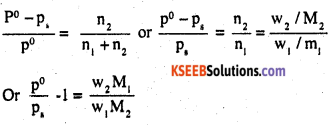substituting the given values, we get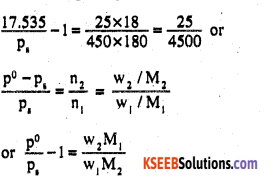substituting the given values, we get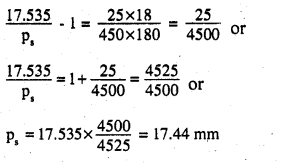Question 35.
Henry’s law constant for the molality of methane in benzene at 298 K is 4.27 x 10s mm Hg. Calculate the solubility of methane in benzene at 298 K under 760 mm Hg.
Here, KH = 4.27 × 105 mm
p = 760 mm
Applying Henry’s law
P = KH x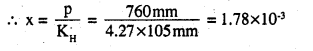i.e, mole fraction of methane in benzene = 1.78 x 10-3

Question 36.
100 g of liquid A (molar mass 140 g mol-1) was dissolved in 1000 g of liquid B (molar mass 180 g mol-1). The vapour pressure of pure liquid B was found to be 500 torr. Calculate the vapour pressure of pure liquid A and its vapour pressure in the solution if the total vapour pressure of the solution is 475 Torr.
Number of moles of a liquid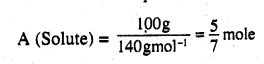Number of moles of a liquid B (Solvent)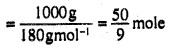Mole fraction of B in the solution (xA)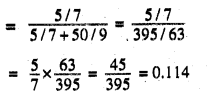Mole fraction of B in the solution (xB)
= 1-0.114 = 0.886
Also, given p0B = 500 Torr
Applying Raoult’s law,
PA = xAA = 0.114 × P°A
PB= xBB = 0.886 × 500 = 443 Torr
p Total = pA + pB
475 = 0.114 P°A +443 or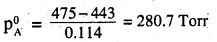Substituting this value in equation (i), we get pA = 0.114x 280.7 Torr = 32 Torr

Question 37.
Benzene and toluene form ideal solution over the entire range of composition. The vapour pressures of pure benzene and toluene at 300 K are 50. 71mm Hg and 32.06 mm Hg respectively. Calculate the mole fraction of benzene in the vapour phase if 80 g of benzene is mixed with 100 g of toluene.
Molar mass of benzene (C6H6 = 78 g mol-1
Molar mass of toluene (C6H5CH3) = 92 g mol-1
∴ Number of moles in 80 g of benzene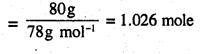Number of moles in 100 g of toluene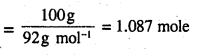In the solution, mole fraction of benzene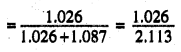=0.486
mole fraction of toluene = 1-0,486 = 0.514
p° Benzene = 50.71 mm, p° Toluene = 32.06mm
Applying Raoult’s law,
p Benzene= xTolucno × p°Tolucne =0.514 × 32.06 mm = 16.48mm
Mole fraction of benzene in vapour phase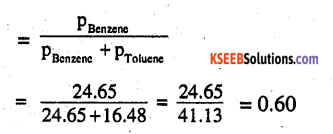Question 38.
The air is a mixture of a number of gases. The major components are oxygen and, nitrogen with approximate proportion of 20% is to 79% by volume at 298 K. The water is in equilibrium with air at a pressure of 10 atm. At 298 K if the Henry’s law constants for oxygen and nitrogen at 298 K are 3.30 × 107 mm and 6.51 × 107 mm respectively, calculate the composition of these gases in water.
Total pressure of air in equilibrium with water = 10 atm
As air contains 20% oxygen and 79% nitrogen by volumes
partial pressure of oxygen :
(P ) = $$\frac{20}{100}$$ x 10 atm = 2atm
= 2 x 760 mm = 1520 mm
partial pressure of nitrogen $$P_{N_{2}}$$
= $$\frac{79}{100}$$ × 10 atm = 7.9 atm = 7.9 x 760 mm
= 6004 mm

KH(O2) = 3.30 x 107mm,KH (N2) = 6.51 × 107 mm
Applying Henry’s law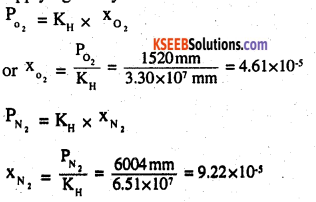Question 39.
Determine the amount of CaCl (i= 2.47) dissolved in 2.51itre of water such that its osmotic pressure is 0.75 atm at 27° C.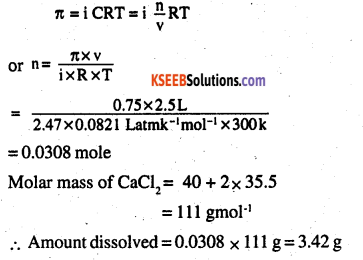Molar mass of CaCl2= 40 + 2x 35.5 = 111 gmol-1
Amount dissolved = 0.0308 xlllg = 3.42g

Question 40.
Determine the osmotic pressure of a solution prepared by dissolving 25 mg of K2SO4in 2 litre of water at 25° C, assuming that it is completely dissociated.
K2SO4 dissolved = 0.025g
volume of solution = 2L
T = 250°C = 298 K
Molar mass of K2SO4 =
2×39 + 32 + 4 × 16= 174gmol-1
As, K2SO4 dissociates completely as K2SO4 → 2K+ SO42-
i.e., ions produced = 3 ∴ i =3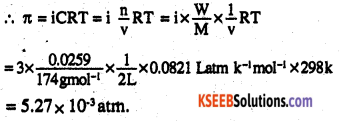Question 1.
How does molarity of a solution change with temperature?
Molarity decreases with increase in temperature because volume of solution increases with increase in temperature.

Question 2.
State Raoult’s law.
Ans:
It states that for a solution of volatile liquids its vapour pressure of each component is directly proportional to their mole fraction in the solution.
The relative lowering of vapour pressure is equal to mole fraction of solute in case of non volatile solute.

Question 3.
Why is liquid ammonia bottle first cooled in ice before opening it?
At room temperature, the vapour pressure of liquid ammonia is very high. On cooling, vapour pressure decreases. Hence the liquid ammonia will not splash out.

Question 4.
Sodium chloride and calcium chloride are used clear show from the roads. Why?
Sodium chloride depresses the freezing point of water to such an extent that it cannot freeze to form ice. Therefore, it melts off easily at the prevailing temperature.

Question 5.
Two liquids A and B boil at 145°C and 190°C
respectively. Which of them has a higher vapour pressure of 80°C? (CBSE 2006)
A being more volatile will have higher vapour pressure at 80°C.Question 6.
Calculate the molality of sulphuric acid solution in which the mole fraction is 0.85.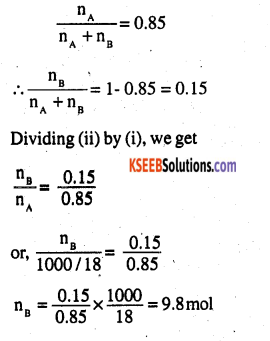Question 7.
The molar freezing point depression constant for benzene is 4.90 kg mol’1. Selenium exists as polymer. When 3.26 g of selenium is dissolved in 226 g of benzene, the observed freezing point is 0.112°C lower for pure benzene. Decide the molecular formula of selenium (At Wt. of selenium is 78.8 g mol-1). (CBSE 2002)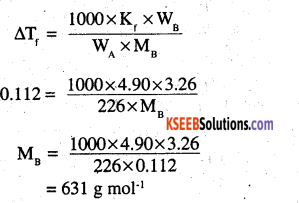Question 8.
CCI4 and water are immiscible whereas ethanol and water are miscible in all proportions. Correlate this behaviour with molecular structure of these compounds. (CBSE 2003,2001)
CCI4 is a non-polar covalent compound.
Water is a polar compound. CCI4 can neither form H bonds with water molecules nor can it break H bonds between water molecules. Therefore, it is insoluble in water.

Ethanol is a polar compound and can form H’ bonds with water, which is a polar solvent, therefore it is miscible with water in all proportions.

Question 9.
The molarity of a solution of sulphuric acid is 1.35 M. Calculate its molarity (The density of the acid solution is 1.02 g cm3 )
Let the solution be 1 litre or 1000 cm3
∴ Number of moles of H2SO4= 1.35
Wt. of solution = 1000 x 1.02 = 1020 g
Wt. of sulphuric acid = 1.35 x 98= 132.3g :
Wt. of water = 1020 – 132.3 = 887.79
Molality of H2SO4= ppp x 1000 = 1.52 m

From (i) M, = 110.82, from (ii) M2 = 196.15 AB4 – AB, = B2 196.15- 110.82 = B2 85.33 = B2 B = 42.665
Molar mass of AB2 = Atomic mass of A+ x 2 atomic mass of B 110.82=Atomic mass of A+85.33 Atomic mass of A = 110.82 – 85.33 = 25.49 Atomic mass of A = 25.499 Atomic mass of B = 42.669

Question 10.
Two elements A and B form compounds having molecular formula AB2 and AB4. When dissolved 20 g of C6H6, 1 g of AB2 lowers the freezing point by 2.3 K, whereas 1.0 g of AB„4 lower it by 1.3 K. The molar depression constant for benzene is 5.1 kg mol*1. Calculate the mass of A and B. (CBSE2004)
Let the molar mass of AB2 and AB4 be M1and M2
Then, for AB2,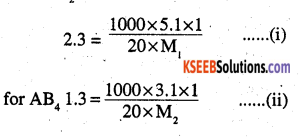From (I) M1 = 110.82, from (ii) M1 = 196.15
AB4– AB2= B2
196.15 – 110.82 =B2
85.33 = B2
B = 42.665
Molar mass of AB2 = Atomic mass of A+ x 2 atomic mass of B
110.82 = Atomic mass of A+ 85.33
∴ Atomic mass of A = 110.82-85.33 = 25.49
Atomic mass of A = 25 .499
Atomic mass of B = 42.669